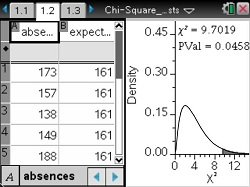### Mathematics lessons for IB® Diploma Programme

Applications and Interpretation | Probability and Statistics## Chi-Squared Tests

### Activity Overview

In this activity, students will look at a problem situation that involves categorical data and will determine which is the appropriate chi-square test to use.

### Objectives

• Students will recognize that chi-squared tests are for counts of categorical data.
• Students will identify the appropriate chi-squared test to use for a given situation: X2 Goodness of Fit Test, X2 Test of Independence, or X2 Test of Homogeneity.
• Students will learn how to calculate the degrees of freedom for each type of chi-squared test.
• Students will interpret the results of a chi-square test.

### Vocabulary

 alpha categorical data chi-squared (X2 distribution) degrees of freedom expected counts goodness-of-fit test observed counts p-value test of homogeneity test of independence